# Theta-series

(diff) ← Older revision | Latest revision (diff) | Newer revision → (diff)-series

A series of functions used in the representation of automorphic forms and functions (cf. Automorphic form; Automorphic function).

Letbe a domain in the complex space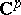,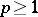, and letbe the discrete group of automorphisms of. Ifis finite, then any function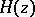,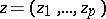, meromorphic ongives rise to an automorphic function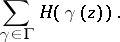For infinite groups one needs convergence multipliers to obtain a theta-series. A Poincaré series, associated to a group, is a series of the form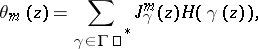(1)

whereis the Jacobian of the function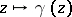andis an integer called the weight or the order. The asterisk means that summation is over those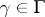which yield distinct terms in the series. Under a mapping,, the function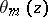is transformed according to the law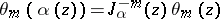, and hence is an automorphic function of weight, associated to. The quotient of two theta-series of the same weight gives an automorphic function.

The theta-series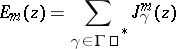is called an Eisenstein theta-series, or simply an Eisenstein series, associated with.

H. Poincaré, in a series of articles in the 1880's, developed the theory of theta-series in connection with the study of automorphic functions of one complex variable. Letbe a discrete Fuchsian group of fractional-linear transformations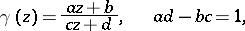mapping the unit disc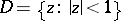onto itself. For this case the Poincaré series has the form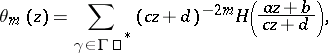(2)

where, for example, is a bounded holomorphic function on. Under the hypothesis thatacts freely onand that the quotient spaceis compact, it has been shown that the series (2) converges absolutely and uniformly onfor. With the stated conditions onand, this assertion holds also for the series (1) in the case whereis a bounded domain in. For certain Fuchsian groups the series (2) converges also for.

The term "theta-series" is also applied to series expansions of theta-functions, which are used in the representation of elliptic functions (cf. Jacobi elliptic functions) and Abelian functions (cf. Theta-function; Abelian function).